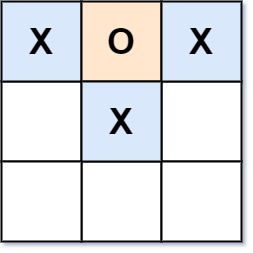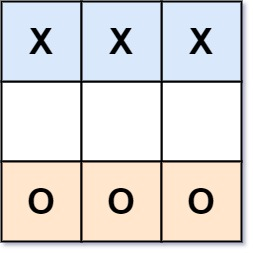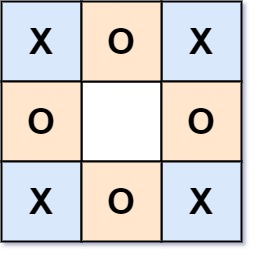0794. Valid Tic Tac Toe State

# 794. Valid Tic-Tac-Toe State#

## 题目 #

Given a Tic-Tac-Toe board as a string array board, return true if and only if it is possible to reach this board position during the course of a valid tic-tac-toe game.

The board is a 3 x 3 array that consists of characters ' ‘, ‘X’, and ‘O’. The ' ' character represents an empty square.

Here are the rules of Tic-Tac-Toe:

• Players take turns placing characters into empty squares ' ‘.
• The first player always places ‘X’ characters, while the second player always places ‘O’ characters.
• ‘X’ and ‘O’ characters are always placed into empty squares, never filled ones.
• The game ends when there are three of the same (non-empty) character filling any row, column, or diagonal.
• The game also ends if all squares are non-empty.
• No more moves can be played if the game is over.

Example 1:``````Input: board = ["O  ","   ","   "]
Output: false
Explanation: The first player always plays "X".
``````

Example 2:``````Input: board = ["XOX"," X ","   "]
Output: false
Explanation: Players take turns making moves.
``````

Example 3:``````Input: board = ["XXX","   ","OOO"]
Output: false
``````

Example 4:``````Input: board = ["XOX","O O","XOX"]
Output: true
``````

Constraints:

• board.length == 3
• board[i].length == 3
• board[i][j] is either ‘X’, ‘O’, or ' ‘.

## 题目大意 #

• 玩家轮流将字符放入空位（’ ‘）中。
• 玩家 1 总是放字符 ‘X’ ，而玩家 2 总是放字符 ‘O’ 。
• ‘X’ 和 ‘O’ 只允许放置在空位中，不允许对已放有字符的位置进行填充。
• 当有 3 个相同（且非空）的字符填充任何行、列或对角线时，游戏结束。
• 当所有位置非空时，也算为游戏结束。
• 如果游戏结束，玩家不允许再放置字符。

## 解题思路 #

• 根据题意棋盘在任意时候，要么 X 的数量比 O 的数量多 1，要么两者相等
• X 的数量等于 O 的数量时,任何行、列或对角线都不会出现 3 个相同的 X
• X 的数量比 O 的数量多 1 时,任何行、列或对角线都不会出现 3 个相同的 O

## 代码 #

``````package leetcode

func validTicTacToe(board []string) bool {
cntX, cntO := 0, 0
for i := range board {
for j := range board[i] {
if board[i][j] == 'X' {
cntX++
} else if board[i][j] == 'O' {
cntO++
}
}
}
if cntX < cntO || cntX > cntO+1 {
return false
}
if cntX == cntO {
return process(board, 'X')
}
return process(board, 'O')
}

func process(board []string, c byte) bool {
//某一行是"ccc"
if board == string([]byte{c, c, c}) || board == string([]byte{c, c, c}) || board == string([]byte{c, c, c}) {
return false
}
//某一列是"ccc"
if (board == c && board == c && board == c) ||
(board == c && board == c && board == c) ||
(board == c && board == c && board == c) {
return false
}
//某一对角线是"ccc"
if (board == c && board == c && board == c) ||
(board == c && board == c && board == c) {
return false
}
return true
}
``````Nov 25, 2022Edit this page